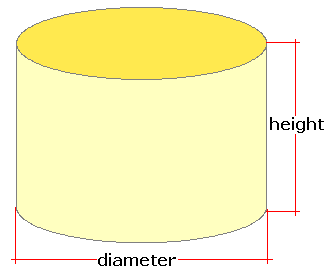Spike's Calculators

# Number of Bushels in a Cylindrical Bin - Metric

Estimate the number of bushels in a round grain bin from measurements in metres. Calculation includes the results for the bin's volume in litres and the bin's capacity in US and Imperial bushels.
The Imperial (Avery) bushel's volume is equal to 36.36872 litres, and the US (Winchester) bushel has a volume of 35.239 litres.##### Volume Formula
```V = Π (D/2)²H;
where V is the volume of the bin
Π = 3.14159
D = the diameter of the bin
H = the height of the bin
```
##### Conversions
```one bushel (bu) Imperial = 36.36872 litres (L)
one bushel (bu) US = 35.23907016688 litres (L)
one litre (L) = 0.0353146667214886 cubic feet (ft³)
```

### Number of Bushels-Grain Bin-Round - Metric

Diameter m
Bin Height m

#### Results:

 Bin Volume in Litres L Bin Volume in Cubic Feet ft³ Imperial Bushels bu Imp US Bushels lbs

#### Calculator

1. diameter of the bin in metres
2. height of the bin in metres

#### Results

1. bin volume in litres
2. bin volume in cubic feet
3. number of Imperial (Avery) bushels
4. number of US (Winchester) bushels